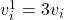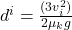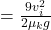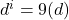## A carton is given a push across a horizontal, frictionless surface. The carton has a mass m, the push gives it an initial speed of vi, and t

Question

A carton is given a push across a horizontal, frictionless surface. The carton has a mass m, the push gives it an initial speed of vi, and the coefficient of kinetic friction between the carton and the surface is μk.
(a) Use energy considerations to find an expression for the distance the carton moves before it stops. (Use any variable or symbol stated above along with the following as necessary)
(b) What if the initial speed of the carton is increased by a factor of 3, determine an expression for the new distance d the box slides in terms of the old distance.

in progress 0
5 months 2021-08-17T07:34:48+00:00 1 Answers 13 views 0

Data provided in the question

Carbon mass = m

Initial speed = v_i

Coefficient = μk

Based on the above information, the expressions are as follows

a. By using the energy considerations the expression for the carton moving distance is

As we know that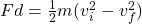where,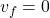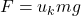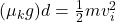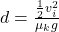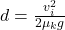b. The initial speed of the carton if the factor of 3 risen, so the expression is